Math & Beyond

# The History of Probability that you need to know

2.3k views

 1 Introduction 2 Etymology 3 Probability in Mathematics 4 Examples of Real-Life probability 5 Summary 6 About Cuemath 7 FAQs 8 External references

7th January 2021

## Introduction

Probability is a measure of the likelihood of an event occuring. It is a branch of Mathematics that studies and analyses the possible outcomes of a given event.

In this article, we will learn about the history and origin of Probability as a stream of Mathematics.

## Etymology

The word Probable refers to the quality or state of being probable.

The word form Probability has its root in,

An old French word called Probabilite

A Latin word Probabilitatem.

## The History of Probability that you need to know - PDF

If you ever want to read it again as many times as you want, here is a downloadable PDF to explore more.

 📥 The History of Probability that you need to know - PDF Download

Probability in Real Life

## Probability in Mathematics

### Origin

The mathematical methods of Probability came into existence around the mid-seventeenth century.

It is said that a dispute between gamblers in the year 1654 led to the birth of Probability as a stream of study in Mathematics. Blaise Pascal and Pierre de Fermat came up with the first set of rules of Probability in Mathematics.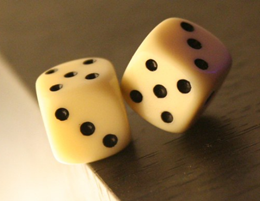A remarkably philosophical French gambler called Chevalier deMéré was curious to find a proper division of stakes when a game of chance was interrupted. He approached Pascal for help, which eventually led to an exchange of thoughts between and Pascal and Fermet.

The outcome of this discussion was the formulation of the fundamental principles of the Theory of Probability.

### Evolution

Christian Huygens, a Dutch scientist, published a book on Probability in 1657 (a first of its kind). The Probability theory gained good popularity and it saw many developments in the 18th century because of contributions from mathematicians like Jakob Bernoulli and Abraham de Moivre. All the techniques developed during this period were concerned with the analysis of games of chance.

Later, in the early 19th century, Pierre de Laplace formulated new techniques and published them in his book Théorie Analytique des Probabilités. The ideas presented here proved useful in solving many practical problems.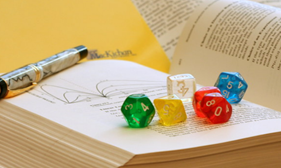Since then, there have been many advancements in this stream of mathematics. Mathematical Statistics is one of the key branches developed based on Probability.

Chebyshev, Markov, von Mises, and Kolmogorov are among the many mathematicians who contributed to this field of study.

## Examples of Real-Life probability

### Tossing a Coin

When a coin is tossed, what is the probability of the outcome being Heads?

Since there are only two possible outcomes (Heads or Tails), there is a 50% chance of the result turning out to be Heads or Tails.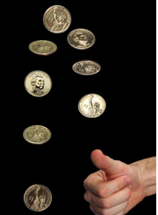### Rolling a Die

Since there are six possible results that can be expected after rolling a die, the probability of seeing a particular number from 1 to 6 is 1 out of 6 (1/6).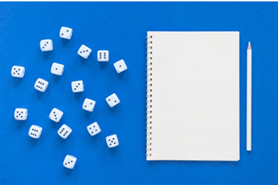### Playing Cards

What is the probability of picking an ace from a deck of cards?

Since there are 52 cards in a deck, the probability of getting one of the four aces is 4 out of 52 (4/52)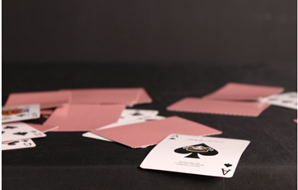### Weather Forecast

Chance of precipitation or Chance of rain for a particular period and place that is reported as part of weather forecasts is an excellent example of the application of probability in our day to day lives.### Forecast of Election results

Political analysts use the technique of Probability to predict the outcome of voting after an election. For instance, they use the results of Exit polls for measuring the Probability.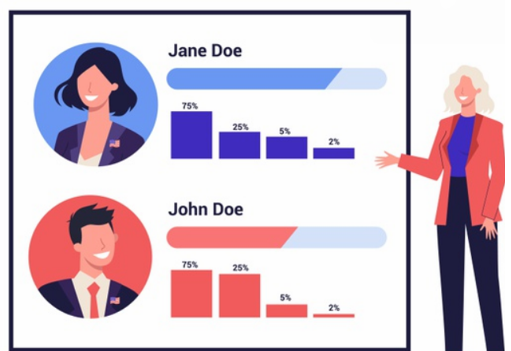## Summary

The scientific formulation of theories of probability is dated back to the 17th century. But similar, albeit less-sophisticated techniques must have been in use much earlier.

We make use of probability every day to make subjective judgment calls and come up with the best possible course of action in any given circumstance.

Probability is, without a doubt, quite indispensable in our daily lives.

### Picture References

School photo created by freepik - www.freepik.com

Cuemath, a student-friendly mathematics and coding platform, conducts regular Online Live Classes for academics and skill-development, and their Mental Math App, on both iOS and Android, is a one-stop solution for kids to develop multiple skills.Understand the Cuemath Fee structure and sign up for a free trial.

## What is probability?

Probability is a branch of Mathematics which deals with the measurement of chances of a particular event happening.

## Who is Blaise Pascal?

Blaise Pascal was a famous French Mathematician, Physicist and a Philosopher. Pascal was responsible for coming up with the foundation for the Theory of Probability along with another famous Mathematician Pierre de Fermet.

## Who is Pierre de Fermat?

Pierre de Fermet was a French Mathematician and a Lawyer. He is the co-founder of the Theory of Probability along with Pascal. He is often called the founder of modern theory of numbers.

## Who is the father of probability theory?

Pierre de Fermet and Blaise Pascal are the founders of the Theory of Probability.

## Who invented probability and statistics?

A dispute during a gambling game led to the invention of the Theory of Probability by the French Mathematicians Pascal and Fermet in the 17th century.

Statistics, as a scientific discipline, came to being in the early 19th century for the purpose of study of populations and economies.